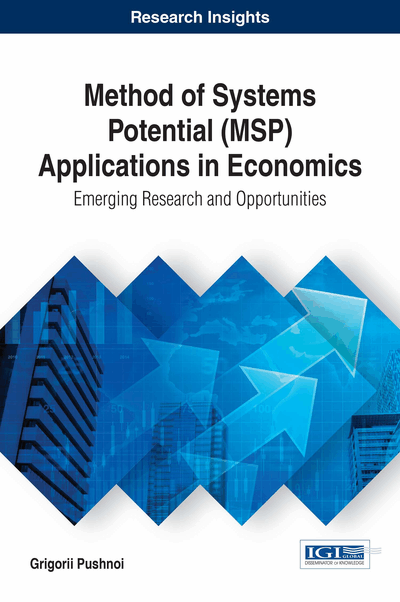# Main Properties of MSP-ECAS-Model: Application to the European Economy

DOI: 10.4018/978-1-5225-2170-9.ch002

Top

## Evolutionary Branches, Long-Term Equilibrium And Temporal Equilibrium States Of Ecas

MSP-modeling opens new possibilities for deeper understanding of the Economic System. MSP-model of ECAS reveals existing implicitly nontrivial economic interrelations between economic indicators. We consider below two cases: 1) MSP-model with constant parameters (Chapter 2 and 3) MSP-model with changing parameters (Chapter 4). Surely MSP-model with constant parameters simplifies of course the economic reality but this model gives important qualitative description of the economy as the complex system which evolves cyclically through a sequence of discontinuous cycles with different durations.

MSP-model with constant parameters introduces into economics new fundamental notion – “evolutionary branch” - locus of temporal equilibrium states of the Economic System in the plane. The fact of existence of such special curves is surprising. We can rewrite equations (19)-(20) from the Introduction for “evolutionary branches” via “productivity of capital”and “capacity utilization rate”.

“Upper Evolutionary Branch” for economic system:

; (1)

“Lower Evolutionary Branch” for economic system:

; (2)

Symboldesignates productivity of capital in the point of long-term equilibrium (Point 1 in Figure 2). The following interrelations are fulfilled:or(3)

Efficiency of economic system depends on productivity of capital (equations (1)-(2)):

“Upper Evolutionary Branch” of economic system:

; (4)

“Lower Evolutionary Branch” of economic system:

; (5)(6)

Parameterinfluences onto the geometric shape of “evolutionary branches”. According to formula (30) from the Introduction this parameter equals to ratio of length of two line segments along Z-axis.

(7)

There are two types of the economic systems:

## Complete Chapter List

Search this Book:
Reset